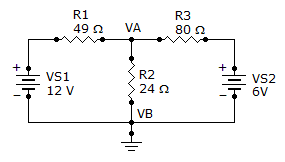# Electrical Engineering - Branch, Loop and Node Analyses - Discussion

Discussion Forum : Branch, Loop and Node Analyses - General Questions (Q.No. 1)
1.
Find the node voltage VA.6 V
12 V
4.25 V
3 V
Explanation:
No answer description is available. Let's discuss.
Discussion:
39 comments Page 2 of 4.

You are right @Krishana.

PRIYANKA said:   6 years ago
According to Kirchofs current law.

Total incoming currents= total outgoing currents.
((12-VA) /49) + ( (6-VA) /80) = (VA/24).
VA=4.25.

Prosper said:   6 years ago
Current flowing through the 49ohms resistor is equal to the sum of current flowing through the 24ohms and 80ohms resistor. eg. I49 = I80 + I24.

Where I49 = (12-VA)/49,
I80 =(VA-6)/80,
I24 = VA/24,
This will give an answer of 2.2945.

Tima said:   6 years ago
What are nodes? Please explain me.

Vikas kumar said:   6 years ago
4.289 volt is the right answer.

Shanawaz said:   6 years ago
Here, we can apply superposition theorem to get the solution.

Neeraj said:   6 years ago
At node vA we can see that Tge current i3 is leaving so the eq must be like,
i1 + i2 - i3 = 0.
(12 - vA)/49 + (6-vA)/80-VA/24 = 0,
Va = 4.2V.

Rj naveed said:   7 years ago
Node analysis can be solved by Kirchhoff's 1st law mean sum of all current toward junction or away from junction is equal to zero.

Saurav said:   7 years ago
Can anyone teach me all the network theorem node analysis?

Abdullah U of R said:   7 years ago
Easy way to calculate node analysis:

i1+i2+i3 = 0 it is equal to:

(12-VA)/49 + -VA/24 + (6-VA)/80 = 0.

12/49 - VA/49 - VA/24 + 6/80 - VA/80 = 0.

12/49 + 6/80 = VA (1/49 + 1/24 + 1/80).

So, VA = (12/49 + 6/80)/(1/49 + 1/24 + 1/80) = 4.28 V.